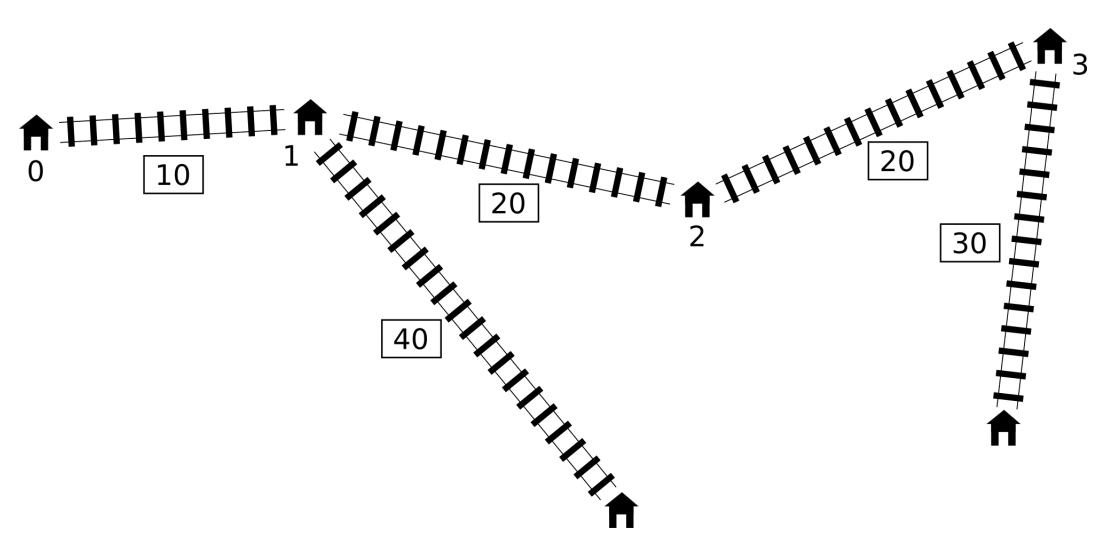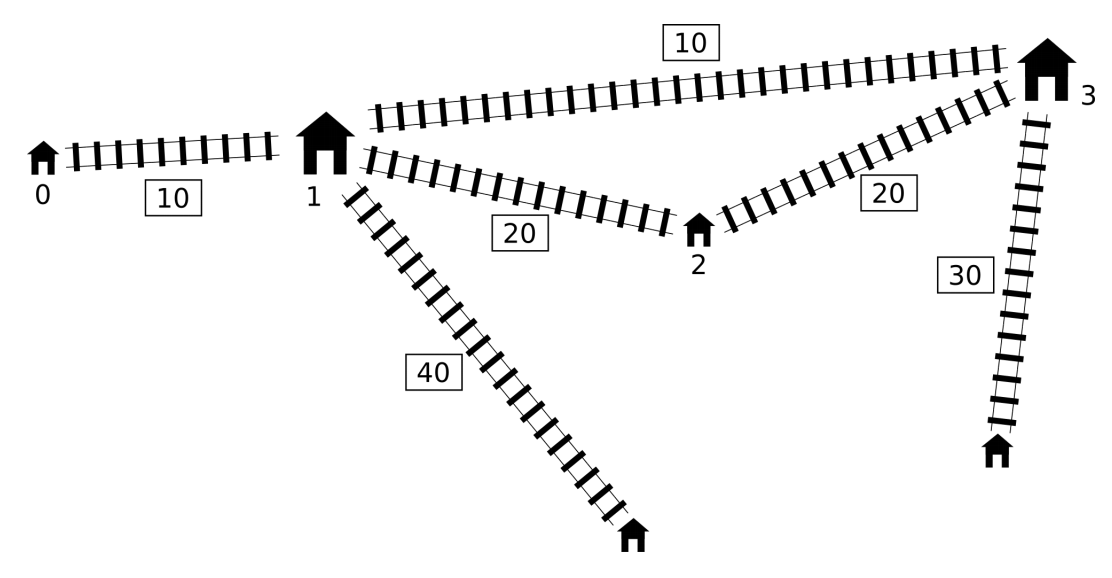시간 제한메모리 제한제출정답맞힌 사람정답 비율
2 초 512 MB139281513.274%

## 문제

Pavel has a toy railway. It is very simple. There is a single main line consisting of n stations. These stations are numbered from 0 to n - 1 in order along the line. The distance between the stations i and i + 1 is li centimeters (0 ≤ in - 1).

Apart from the main line there may be some secondary lines. Each secondary line is a railway line between a station on the main line and a new station that does not lie on the main line. (These new stations are not numbered.) At most one secondary line can start in each station of the main line. The length of the secondary line starting at station i is di centimeters. We use di = 0 to denote that there is no secondary line starting at station i.Pavel is now planning to build one shortcut: an express line between two different (possibly neighbouring) stations of the main line. Express line will have length of exactly c centimeters, regardless of what two stations it will connect.

Each segment of the railway, including the new express line, can be used in both directions. The distance between two stations is the smallest length of a route that goes from one station to the other along the railways. The diameter of the whole railway network is the maximum distance among all pairs of stations. In other words, this is the smallest number t, such that the distance between every pair of stations is at most t.

Pavel wants to build the express line in such a way that the diameter of the resulting network is minimized.

## 구현

You should implement the function

• int64 find_shortcut(int n, int[] l, int[] d, int c)
• n: number of stations on the main line,
• l: distances between stations on the main line (array of length n - 1),
• d: lengths of secondary lines (array of length n),
• c: length of the new express line.
• the function should return the smallest possible diameter of the railway network after adding the express line.

## 예제 1

For the railway network shown above, the grader would make the following function call:

find_shortcut(4, [10, 20, 20], [0, 40, 0, 30], 10)

The optimal solution is to build the express line between stations 1 and 3, as shown below.The diameter of the new railway network is 80 centimeters, so the function should return 80.

## 예제 2

The grader makes the following function call:

find_shortcut(9, [10, 10, 10, 10, 10, 10, 10, 10], [20, 0, 30, 0, 0, 40, 0, 40, 0], 30)

The optimal solution is to connect stations 2 and 7, in which case the diameter is 110.

## 예제 3

The grader makes the following function call:

find_shortcut(4, [2, 2, 2], [1, 10, 10, 1], 1)

The optimal solution is to connect stations 1 and 2, reducing the diameter to 21.

## 예제 4

The grader makes the following function call:

find_shortcut(3, [1, 1], [1, 1, 1], 3)

Connecting any two stations with the express line of length 3 does not improve the initial diameter of the railway network which is 4.

## 제한

In all Subtasks 2 ≤ n ≤ 1,000,000, 1 ≤ li ≤ 109, 0 ≤ di ≤ 109, 1 ≤ c ≤ 109.

## 서브태스크

번호배점제한
19

2 ≤ n ≤ 10

214

2 ≤ n ≤ 100

38

2 ≤ n ≤ 250

47

2 ≤ n ≤ 500

533

2 ≤ n ≤ 3,000

622

2 ≤ n ≤ 100,000

74

2 ≤ n ≤ 300,000

83

2 ≤ n ≤ 1,000,000

## 샘플 그레이더

The sample grader reads the input in the following format:

• line 1: integers n and c,
• line 2: integers l0, l1, ..., ln-2,
• line 3: integers d0, d1, ..., dn-2.

## 제출할 수 있는 언어

C++17, C++14, C++20, C++14 (Clang), C++17 (Clang), C++20 (Clang)

## 채점 및 기타 정보

• 예제는 채점하지 않는다.